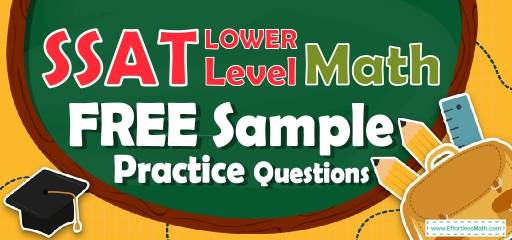# SSAT Lower Level Math FREE Sample Practice QuestionsPreparing your student for the SSAT Lower Level Math test? To do their best on the SSAT Lower Level Math test, students need to review and practice real SSAT Lower Level Math questions.  There’s nothing like working on SSAT Lower Level Math sample questions to hone your student’s math skills and put them more at ease when taking the SSAT Lower Level Math test. The sample math questions you’ll find here are brief samples designed to give students the insights they need to be as prepared as possible for their SSAT Lower Level Math test.

Check out our sample SSAT Lower Level Math practice questions to find out what areas your student needs to practice more before taking the SSAT Lower Level Math test!

Help your students start preparing for the 2022 SSAT Lower Level Math test with our free sample practice questions. Also, make sure to follow some of the related links at the bottom of this post to get a better idea of what kind of mathematics questions your students need to practice.

## 10 Sample SSAT Lower Level Math Practice Questions

1- What is the next number in this sequence? $$2, 5, 9, 14, 20, …$$

A. 27

B. 26

C. 25

D. 21

E. 20

2- What is the average of the following numbers? $$6, 10, 12, 23, 45$$

A. 19

B. 19.2

C. 19.5

D. 20

E. 25

3- If there are 8 red balls and 12 blue balls in a basket, what is the probability that John will pick out a red ball from the basket?

A. $$\frac{18}{20}$$

B. $$\frac{2}{5}$$

C. $$\frac{2}{10}$$

D. $$\frac{3}{5}$$

E. $$\frac{20}{10}$$

4- How many lines of symmetry do an equilateral triangle have?

A. 5

B. 4

C. 3

D. 2

E. 1

5- What is $$\%10$$ of 200?

A. 10

B. 20

C. 30

D. 40

E. 50

6- At a Zoo, the ratio of lions to tigers is 5 to 3. Which of the following could NOT be the total number of lions and tigers in the zoo?

A. 64

B. 80

C. 98

D. 104

E. 160

7- In the multiplication bellow, A represents which digit?
$$14×3?2=4,788$$

A. 2

B. 3

C. 4

D. 6

E. 8

8- Four people can paint 4 houses in 10 days. How many people are needed to paint 8 houses in 5 days?

A. 6

B. 8

C. 12

D. 16

E. 20

9- $$8.9−4.08$$ is closest to which of the following.

A. 4.1

B. 4.8

C. 6

D. 8

E. 13

10- $$x=2,456 \ y=259$$
Numbers $$x$$ and $$y$$ are shown above. How many times larger is the value of digit 5 in the number $$x$$, than the value of digit 5 in the number $$y$$?

A. 1

B. 10

C. 100

D. 1,000

E. 10,000

## BestSSAT Lower Level Math Prep Resource for 2022

1- A
$$2+3=5→5+4=9→9+5=14→14+6=20→20+7=27$$

2- B
average$$=\frac{(sum \ of \ all \ numbers)}{(number \ of \ numbers)}=\frac{(6+10+12+23+45)}{5}=19.2$$

3- B
There is 8 red ball and 20 are a total number of balls. Therefore, the probability that John will pick out a red ball from the basket is 8 out of 20 or $$\frac{8}{(8+12)}=\frac{8}{20}=\frac{2}{5}$$.

4- C
An equilateral triangle has 3 lines of symmetry.

5- B
10 percent of $$200 = 10\%$$ of $$200 = \frac{10}{100}×200=20$$

6- C
The ratio of lions to tigers is 5 to 3 at the zoo. Therefore, the total number of lions and tigers must be divisible by 8.
$$5+3=8$$
From the numbers provided, only 98 is not divisible by 8.

7- C
A represents digit 4 in the multiplication.
$$14×342=4,788$$
Only choice C follows the rule of adding 3 in the sequences.

8- D
Four people can paint 4 houses in 10 days. It means that for painting 8 houses in 10 days we need 8 people. To paint 8 houses in 5 days, 16 people are needed.

9- B
$$8.9-4.08=4.82$$, which is closest to 4.8

10- A
The value of digit 5 in both numbers $$x$$ and $$y$$ are in the tens place. Therefore, they have the same value.

Looking for the best resource to help you succeed on the SSAT Lower Level Math test?

## The Best Books to Ace the SSAT Lower Level Math Test

### What people say about "SSAT Lower Level Math FREE Sample Practice Questions - Effortless Math: We Help Students Learn to LOVE Mathematics"?

No one replied yet.

X
30% OFF

Limited time only!

Save Over 30%

SAVE $5 It was$16.99 now it is \$11.99